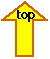### ATMS 749 HOMEWORK {HW1, HW2, HW3, HW4,HW5, HW6, HW7, HW8} {HW Class of 2011} [Main Page] [Daily Notes]

HINTS ON HOMEWORK:
MAKE A SKETCH OF THE PROBLEM SET UP WHENEVER POSSIBLE!
Homework problems are intended to help you master the course material. You are strongly encouraged to work with others, but make your submitted homework a unique expression of your knowledge of the material.
A more thorough discussion of this process is given in the course description.

Example 1 of an easy to read, clear homework page. (Nice handwriting with a pen, on one side of the page only).
Example 2 of a word-processed homework page.

ASSIGNMENT 8 (see calendar for due date).

1. Comparing the lifecyle of stars like our sun, Carl Sagan pointed out that the solar output was likely only about 70% of the current
value in the Earth's early history. This is known as the faint young sun paradox because it is likely that liquid water was present at this time, rather than ice.
What was the wavelength of maximum emission for the early sun?
What is a likely scenario for the composition of the Earth's early atmosphere that resolves this paradox?

2. How are lasers and light emitting diodes different from blackbody emitters?

3. What effect do clouds have on the surface cooling rate at night?

4. Problem 6.5.

5. Calculate and plot the atmosphere temperature and surface temperature as a function of atmospheric absorption from 0 to 1.
Use the long wave absorptivity = 0.8, and the surface albedo = 0.3. Interpret. Is increasing atmospheric absorptivity a viable
way of decreasing surface temperature (does it work? Is it a good idea? What are consequences?)

6. Problem 6.28.

ASSIGNMENT 7 (see calendar for due date).

In this assignment you'll make graphs and interpret them (not just provide numbers).

This is a long assignment so get started early.

The first 4 problems help you understand how to set up problem 5.

Problems 1- 4. As described here.

Problem 5. See the presentation for the 2 stream model for this problem.
Note: This problem teaches you how to also solve the problem of snow albedo with embedded aerosol,
and the Aethalometer (filter based light absorption measurement and model.)

Aerosol Indirect Effect

Reproduce the figure below using the simple model for reflectance with absorption values
of SSA=1 and SSA≠1 where SSA is single scatter albedo. Calculate the cloud albedo as a
funciton of effective radius and liquid water path for single scattering albedo equal to 1.0 and 0.95.
In the second part for SSA=0.95, assume that the absorption is caused by black carbon aerosol
embedded in the cloud. Calculate the absorption optical depth necessary to give the value
of single scattering albedo as a function of the liquid water patth. Take the black carbon aerosol
single scattering albedo to be 0.4. The cloud droplet asymmetry parameter is 0.85. Comment
on the likelihood of observing these absorption coefficients. Finally, comment on now aerosol
light absorption impacts the aerosol indirect effect (i.e. the increased cloud albedo
because of smaller more numerous droplets.)

(Here is a hint on how to do the absorption optical depth calculation).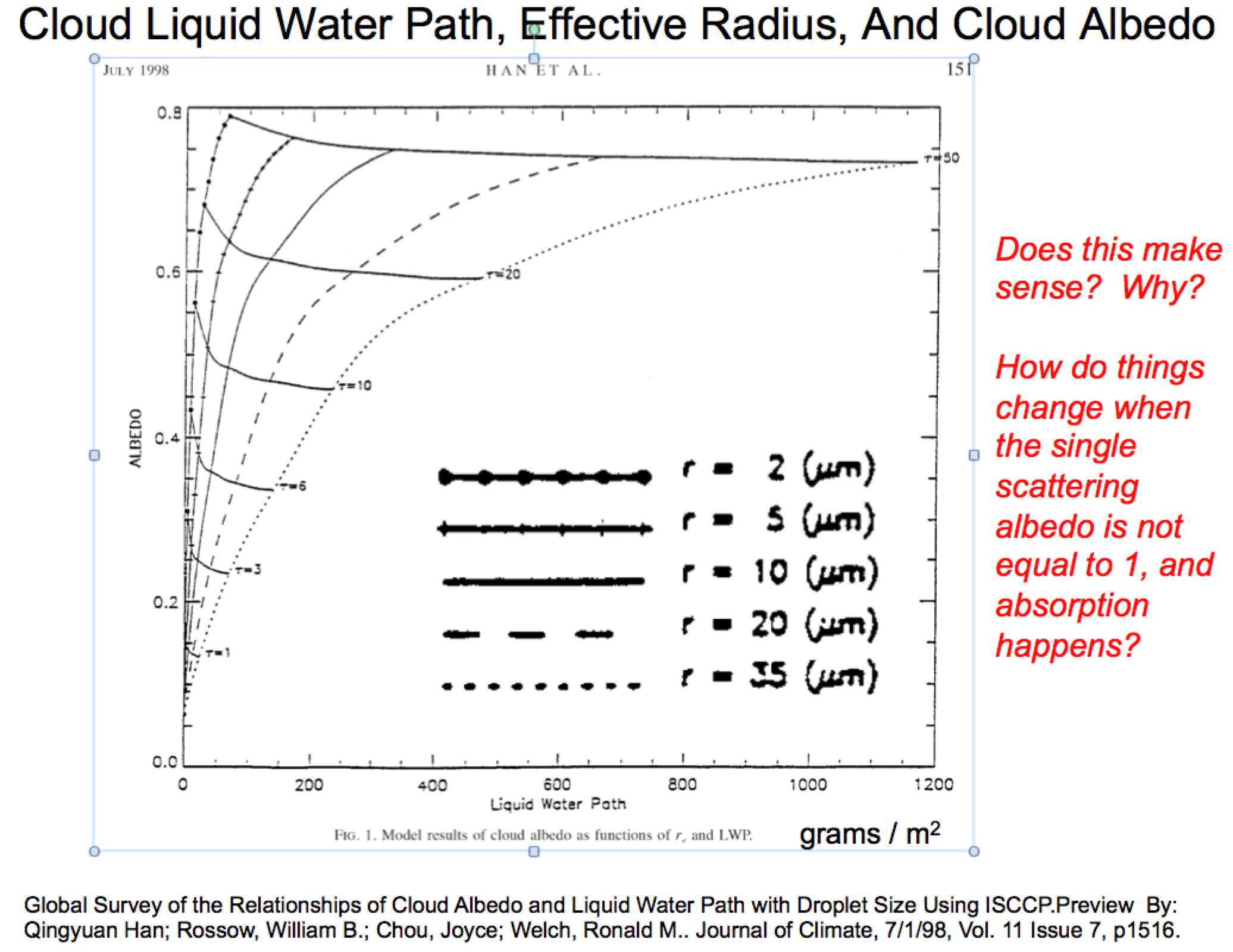Problem 6

Calculate dR/dCCN from the conservative case (single scattering albedo = 1) and interpret. Use of the chain rule for differentiation
and the results of the last problem in HW5 help with this problem.

ASSIGNMENT 6 (see calendar for due date).

Read chapter 11 and Appendix A, and chapter 11 notes may be helpful.

Problem 1:
Determine the Legendre polynomial expansion coefficients Beta0, Beta1, and Beta2 for the Rayleigh phase function.

Problem 2:
Use the two term phase function p[cos(theta)]=(1 + 3gcos(theta)) to calculate the downwelling irradiance in the single scattering
approximation as we did for the Rayleigh phase function in class [Use the small optical depth approximation]. Interpret your results.

ASSIGNMENT 5 (see calendar for due date).

Do problems (in this order):

7.4

7.8 Sunphotometer problem, describes how optical depth is obtained experimentally.
Note that usually more than two points are used, as illustrated in the 'Langley plot' of
figure 7.9

7.10

7.12

7.11 Cloud-aerosol interaction, aerosol indirect effect. part a) is the problem listed in the book.
The derivation is easy if you assume all cloud droplets have the same radius.
However, for a polydispersion, use of the mean volume radius to represent the mean area radius
is an assumption that is not generally viable.
7.11 part b) as described next: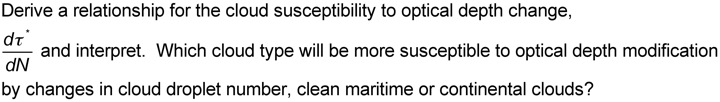7.13

ASSIGNMENT 4 (see calendar for due date).

INSTRUCTIONS:
You can choose either of the problem 1 to do (you don't HAVE to do both). Everyone will do problems 2 through 4.
You CAN do both problem 1 and treat one as extra credit. If you are doing a lot of research in aerosol optics, it would be good
to do first problem 1. Ask me if you have any questions.

1. Reno experienced a brief dust storm in the afternoon of 24 April 2013.
The UNR Cimel sunphotometer observed the following optical depth time series on this day.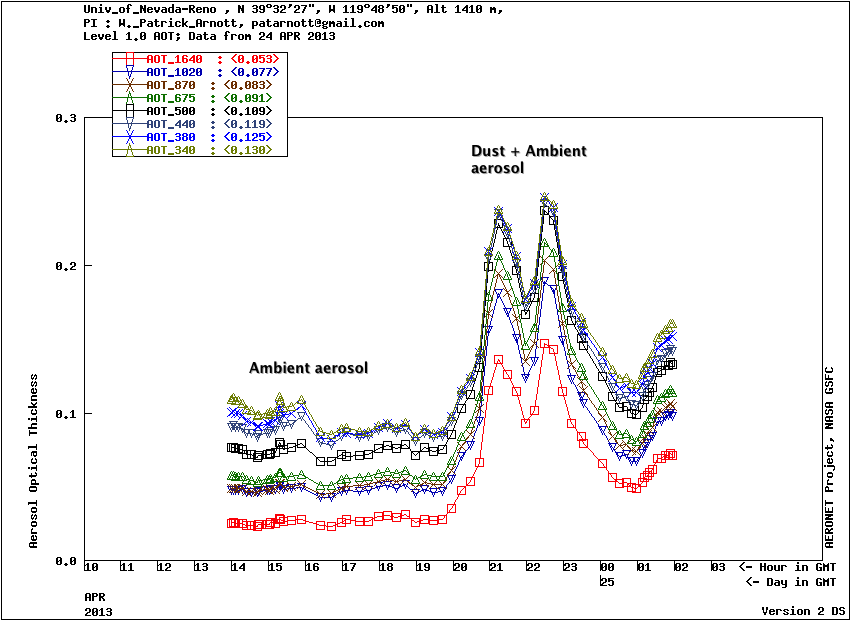Figure 1.1. Dust from the Carson Sink blew over Reno on 24 April 2013.
Click on the figure to see a larger version. The optical depth was measured
with the UNR Cimel sunphotometer as part of the NASA AERONET network.

A retrieved size distribution (by Dambar Air, using MFRSR data) for the dust case
is shown in Figure 1.2 along with the size distribution for the Rim Fire case for comparison.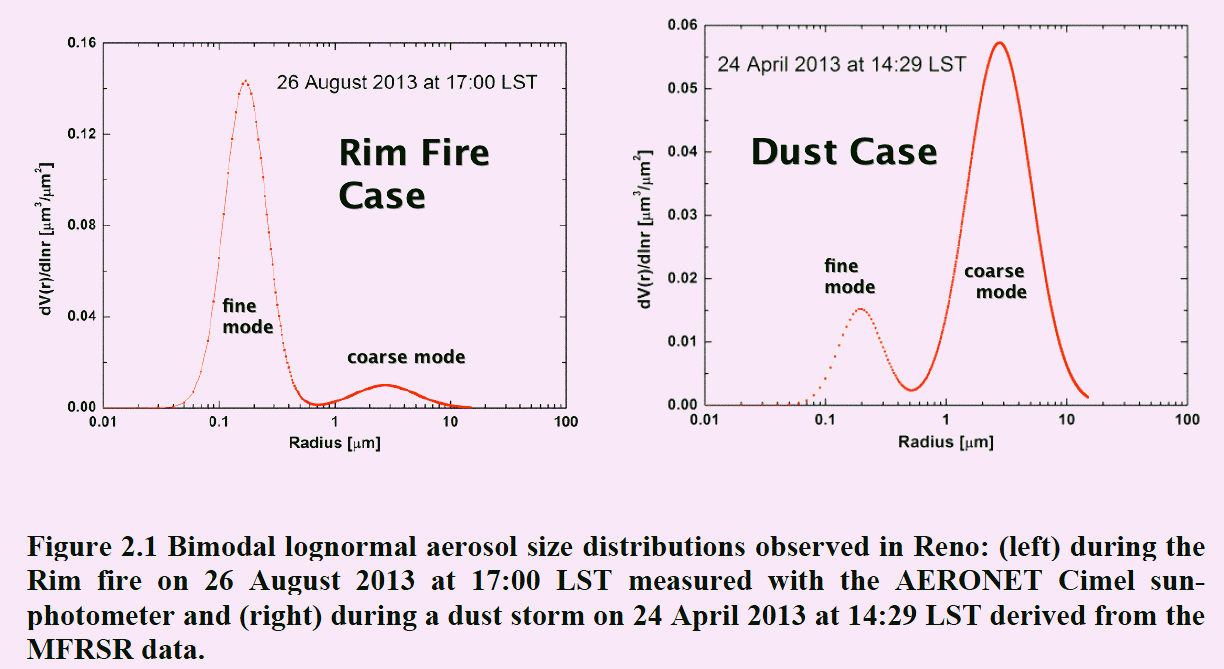Figure 1.2. Rim fire and dust case study size distributions. Click on image for larger version.

The column averaged volume distribution is shown in Figure 1.2.
The size distribution is the sum of two lognormal distributions,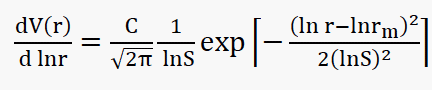.
The table of lognormal parameters for the two cases is shown here. Note the dominance
of the fine mode for the Rim fire and the coarse mode for the dust case.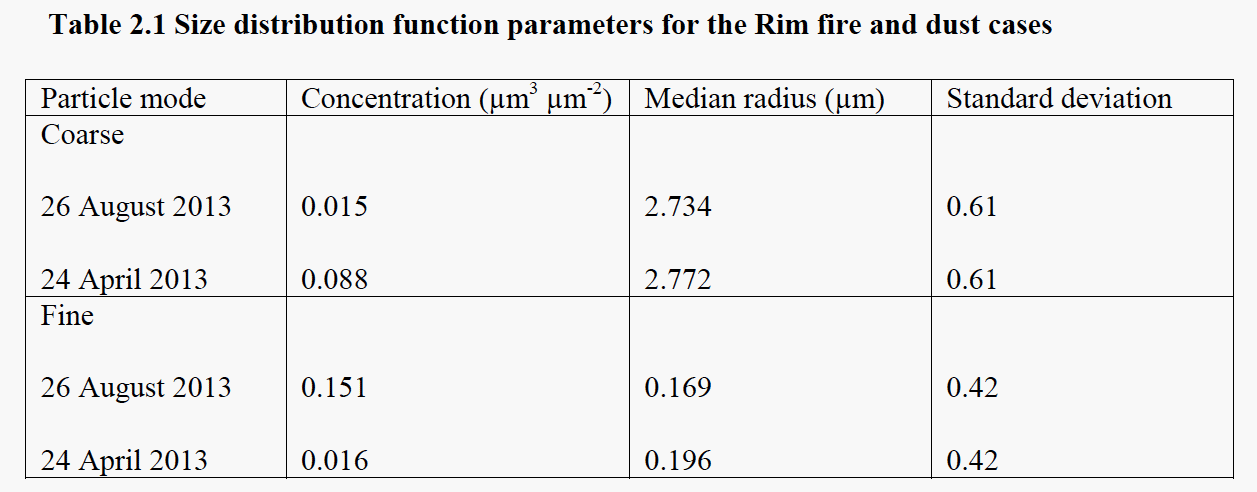For this problem, calculate the fine and coarse mode optical depth at 405 nm and 870 nm, and the total optical depth,
for the dust case and the Rim fire case. The theory you need to use is summarized here. You will need to find a Mie theory code
to compute Qext(wavelength, radius) to do the integration over aerosol size distribution.
Mie code (BHMIE by Bohren and Huffman) in Fortran, C, Matlab, and Python can be found here.
You'll have to make a choice for refractive index for each mode - explain your choice -
here is a paper that reports some refractive index measurements for dust;
(this quantity is often retrieved during the Cimel analysis.)
Discuss the relative contribution of the fine and coarse modes to the extinction at 405 nm and 870 nm.
Also compute to asymmetry parameter for each size mode, and the total, for each wavelength, and discuss.
The extinction optical depth and the asymmetry parameter are needed to do radiative forcing estimates,
and are needed for 2 stream models of multiple scattering theory.

A summary of the theory is next: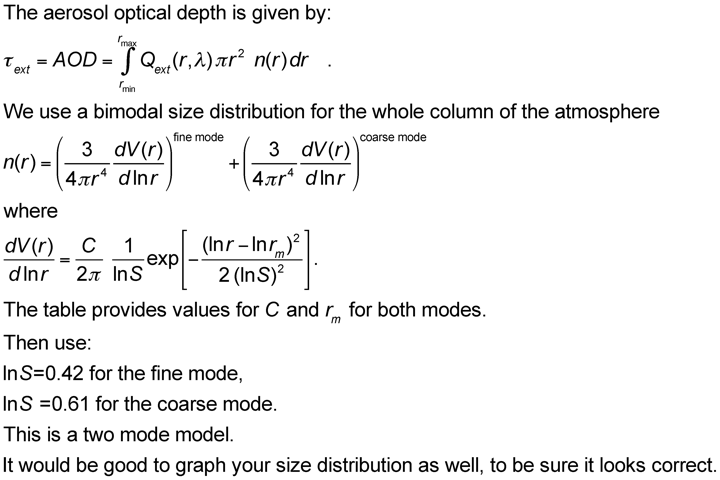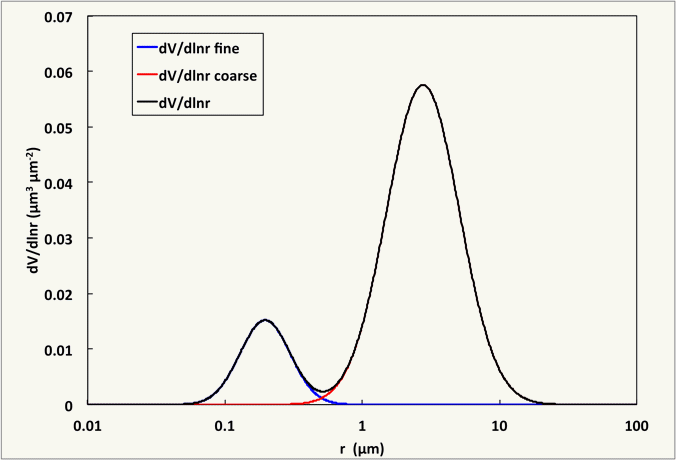Here is the dust case size distribution for reference, and an excel spreadsheet for the bimodal distribution.

1. Use analytical expressions for the scattering and absorption efficiencies for parts a. and b.
a. Assuming the size parameter, x, is much less than 1 (x<<1), what is ratio of the total scattering cross section of a sphere having
complex refractive index (1.5,0.5) (put this in the numerator) with that of a sphere having complex refractive index (1.5,0.00000005) (put this in the denominator)?
The first refractive index is roughly that of black carbon; the second is that of ammonium sulfate; both for the visible wavelength range.
b. Do the same calculation as in a.) but for now for the total absorption cross section of these two spheres.
c. Now discuss your results in parts a. and b. Can you say that a strong absorber is a weak scatterer?

The Mie theory calculator may be used for the rest of the problem.

NOTE:
To obtain enough precision for Qext and Qsca to obtain Qabs by subtraction,
you'll have to use complex refractive index (1.5,0.000005) for ammonium sulfate for the remaining parts of this problem.

You can choose a wavelength (say 0.5 microns) and calculate the sphere diameter, using the given size parameter, to use with the Mie theory calculator.
d. Repeat part a. for a size parameter of 4.6.
e. Repeat part b. for a size parameter of 4.6.
f. Discuss your results as in part c. What scattering regime is this?
g. Repeat part a. for a size parameter of 100. Choose an aerosol concentration of 0.0001 to avoid concentration issues. (This choice does not affect the Q calculations).
h. Repeat part b. for a size parameter of 100.
i. Discuss your results as in part c. What scattering regime is this?

It may be most clear to put the results of parts a-i in a single table, and then to discuss the table.

j. Above what size parameter does the small particle approximation in parts a-c produce an error for scattering and absorption in excess of 10%?

2. This problem is discussed in the presentation for chapter 12.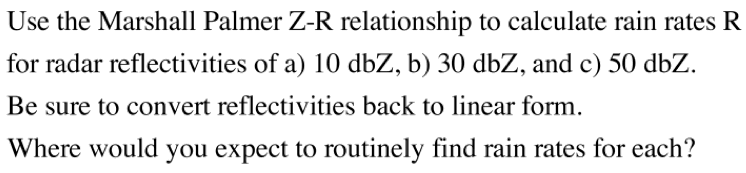3. Problem 12.7.

4. Problem 12.8.

ASSIGNMENT 3 (see calendar for due date).

1. Download the latest compilation of the complex refractive index for ice from here, or locally from here. Then calculate and interpret the following:
a) Absorption coefficient.
b) Penetration depth.
c) Reflection coefficient for normal incidence.
d) Reflection coefficient for 45 degree incidence (both polarizations and the average of them).
Hint: You might wish to solve this problem first and use its results, or just use complex variables in Excel or Fortran, etc.
e) Brewster angle.

2. Soot is the colloquial name for the black aerosol that spews from poorly regulated engine exhausts. The refractive index of soot likely varies with the details of the combustion process, but is roughly N=(1.75,0.75i) at around 550 nm in the green part of the electromagnetic spectrum. Calculate the reflectivity of a slab of soot at normal incidence. Compare with the reflectivity of water at normal incidence where the refractive index is N=(1.33, 1x10-7). Compare the penetration depth of light in each of these substances. Is it correct that a slab of soot has a reflection coefficient at normal incidence that's greater than that of water, afterall, we also call soot 'black carbon' because it is so dark. Interpret.

3. Suppose you have a customer that wants you to build in instrument for light absorption that they want to use to also measure black carbon mass concentration. Your customer has soot particles that, miraculously, are perfect spheres in 3 diameters: 30 nm, 100 nm, and 250 nm. Using the refractive index for soot at 550 nm given in the presentation for chapter 2, calculate the wave penetration depth at this wavelength. Would this wavelength be a good choice to provide measurements of the black carbon mass concentration for this customer for all soot diameters? If not, choose a wavelength from the list of available lasers at 355 nm, 405 nm, 532 nm, 870 nm, and 1047 nm to use for the light absorption measurements that would work better for the black carbon mass concentration measurement for all soot diameters (you can make the unjustified but expedient assumption that the refractive index remains the same at all of these wavelengths and at 550 nm).

4. Derive an expression for the rainbow angle of a spherical particle as a function of the refractive index (assumed real only). You can do this by solving the condition db/dTheta = 0 (see figures 4.7 and 4.8.) Here b is the impact parameter equal to the sphere radius * sin(thetai). Use your solution to discuss features of the rainbow including for water (rain drops or cloud droplets) why we see color, and why the rainbow is bright. What condition on the refractive index must happen so that the rainbow angle is associated with exact backscattering (this represents the merging of the glory and the rainbow)? Hint: This link is useful. You are looking for a relationship between the scattering angle and the refractive index for the rainbow ray on the cover of the text book.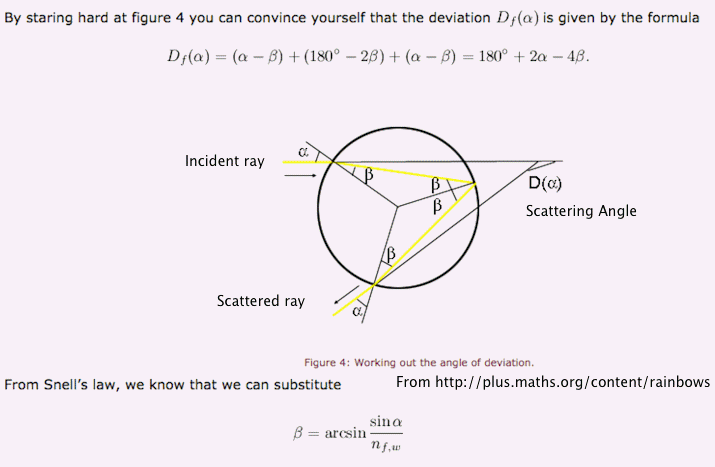Here is another point of view (from this link).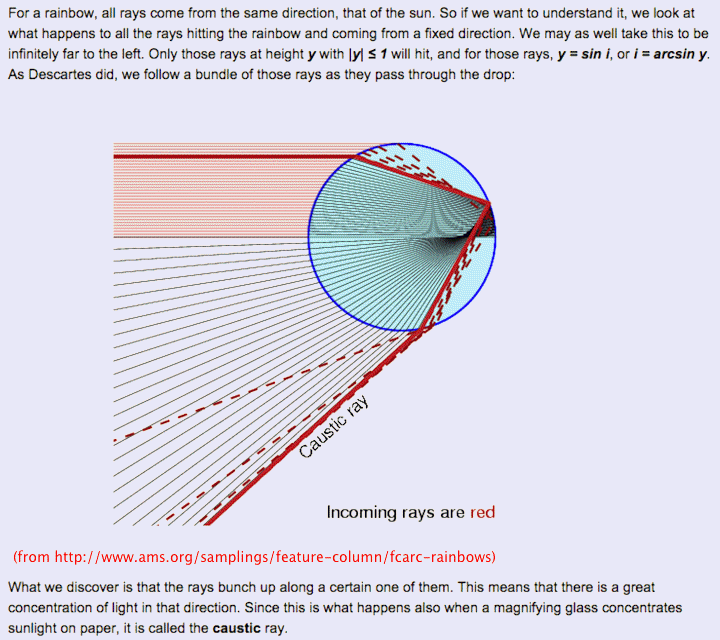Here is another link that may be useful. The image below is from this link. It shows the electromagnetic field in the vicinity of the droplet. Click on the image (or here) to see an animated version.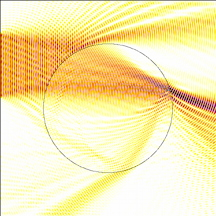Here is another ray diagram that may be useful.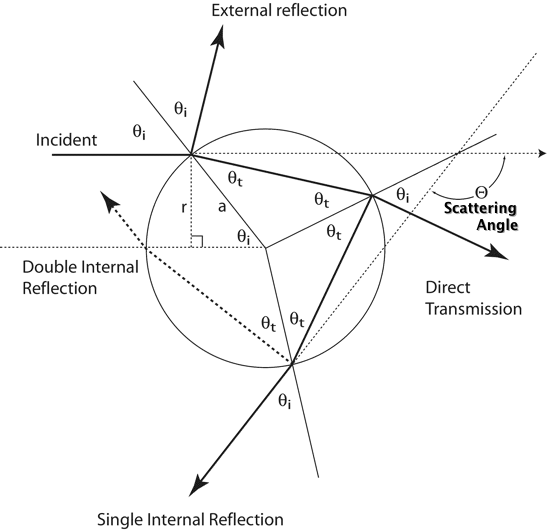Extra Credit: (due any time during the semester).
4. Repeat problem 1 for water (refractive index table , and fortran code for the same).

5. Repeat problem 2, though for ice and atmospheric halos and sun dogs.

If you like these subjects, you can study these papers related to wave propagation in complex media to get more of the story:
Ray tracing, general theory, total internal reflection, quick discussion.

ASSIGNMENT 2 due September 11th before class.

Problems 2.7, 2.8, 2.9, 2.10, 2.12, 2.14, 2.20. Also, define and discuss actinic flux; why is it important?

The approximation for small solid angle is discussed here: that will probably be useful for some problems.

ASSIGNMENT 1 due September 2nd before class.

Read chapters 1 and 2. Problems 1.1, 1.2, 2.1, 2.2 and 2.3. On problem 2.1, add a part d) as follows. 2.1 d) What is the wavelength of a microwave oven, and why is it that wavelength?